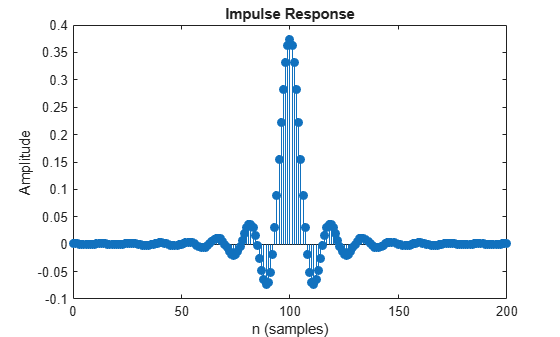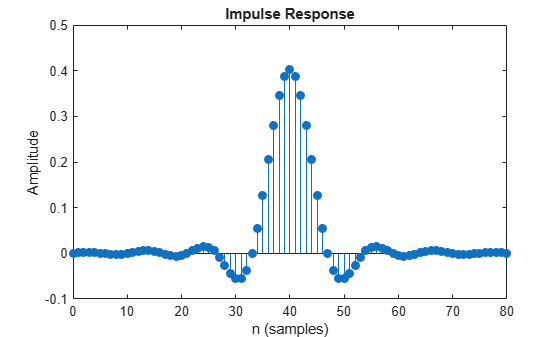# coeffs

Coefficients for filters

## Syntax

``coefInfo = coeffs(rcfilter)``
``coefInfo = coeffs(rcfilter,'Arithmetic',arithType)``

## Description

````coefInfo = coeffs(rcfilter)` obtain the coefficients for the specified filter System object™.```

example

````coefInfo = coeffs(rcfilter,'Arithmetic',arithType)` analyzes the filter System object based on the arithmetic specified in `arithType`.```

## Examples

collapse all

Create a receive raised cosine filter and obtain its numerator coefficients.

```rxfilter = comm.RaisedCosineReceiveFilter('FilterSpanInSymbols',25); srx = coeffs(rxfilter)```
```srx = struct with fields: Numerator: [1x201 double] ```

Calculate the expected number of numerator coefficients and confirm the value equals the length of `srx.Numerator`.

`numcoefs = rxfilter.FilterSpanInSymbols * rxfilter.InputSamplesPerSymbol + 1`
```numcoefs = 201 ```
`isequal (numcoefs,length(srx.Numerator))`
```ans = logical 1 ```

Display the first ten coefficients.

`srx.Numerator(1:10)`
```ans = 1×10 10-3 × 0.9094 0.8559 0.6136 0.2320 -0.2074 -0.6071 -0.8759 -0.9486 -0.8021 -0.4623 ```

Display the impulse response of the receive raised cosine filter.

`fvtool(rxfilter,'impulse')`Create a transmit raised cosine filter and obtain its numerator coefficients.

```txfilter = comm.RaisedCosineTransmitFilter('RolloffFactor',0.5); stx = coeffs(txfilter);```

Display the first ten filter coefficients.

`stx.Numerator(1:10)`
```ans = 1×10 -0.0002 0.0011 0.0021 0.0024 0.0018 0.0004 -0.0014 -0.0029 -0.0036 -0.0031 ```

Display the impulse response of the transmit raised cosine filter.

`fvtool(txfilter,'impulse')`## Input Arguments

collapse all

Input filter, specified as one of these of filter System objects.

Specify the arithmetic used in the analysis. When the arithmetic input is not specified and the filter System object is unlocked, the analysis tool assumes a double-precision filter. When the arithmetic input is not specified and the System object is locked, the function performs the analysis based on the data type of the locked input.

The `'Arithmetic'` input argument specified as `'Fixed'` applies only to filter System objects with fixed-point properties.

When the `'Arithmetic'` input argument is specified as `'Fixed'` and the filter object has coefficients data type set to `'Same word length as input'`, the arithmetic analysis depends on whether the System object is:

• `unlocked` –– The analysis object function cannot determine the coefficients data type. The function assumes that the coefficients data type is signed, has 16-bit word length, and is auto scaled. The function performs fixed-point analysis based on this assumption.

• `locked` –– When the input data type is `'double'` or `'single'`, the analysis object function cannot determine the coefficients data type. The function assumes that the coefficient data type is signed, has 16-bit word length, and is auto scaled. The function performs fixed-point analysis based on this assumption.

To check if the System object is locked or unlocked, use the `isLocked` function.

When the arithmetic input is specified as `'Fixed'` and the filter object has coefficients data type set to a custom numeric type, the object function performs fixed-point analysis based on the custom numeric data type.

## Output Arguments

collapse all

Filter coefficient info, returned as a structure containing the filter coefficients in the `Numerator` field. When the filter uses fixed-point arithmetic, the function returns additional information about the filter. This information includes the arithmetic setting and details about the filter internals.

### Objects

Introduced in R2013b